Data Analyzed By: Lusi Yang @ Corning Inc.

# Analyze the AAPL Stock¶

In :
# Load up the required packages
library(MASS)
library(quantmod)

In :
# Load up Apple's Stock AAPL
getSymbols("AAPL",src="yahoo")

'AAPL'
In :
# Read the column names
colnames(AAPL)

1. 'AAPL.Open'
2. 'AAPL.High'
3. 'AAPL.Low'
4. 'AAPL.Close'
5. 'AAPL.Volume'
In :
# Visualize the AAPL Stock
barChart(AAPL)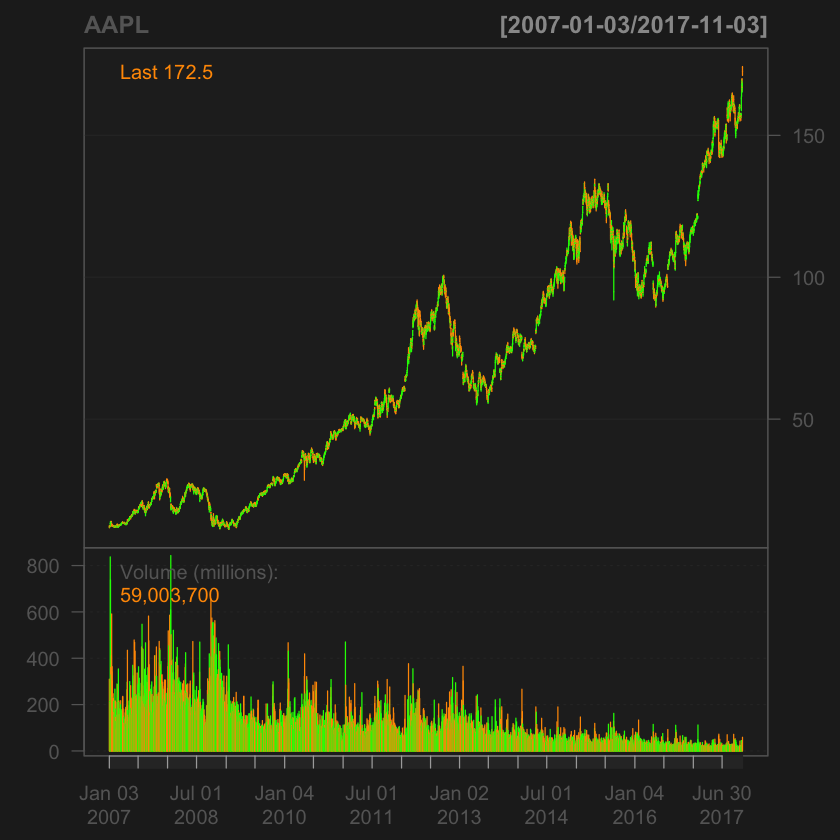Apple's stock is going up over time, but it is definetely not stationary. However, in recent time, Apple's stock volume in trade has been declining as iPhone 8 & 8 Plus was introduced to the public. This decline indicates that investors are losing faith in Apple; thus, Apple stock has become less active than before.

Stationarity

In :
# The original series is not stationary, so it needs differencing
par(mfrow=c(2,1))
acf(as.ts(AdjClosed), main = "Autocorrelation of AAPL")
acf(diff(as.ts(AdjClosed)), main = "Autocorrelation of AAPL")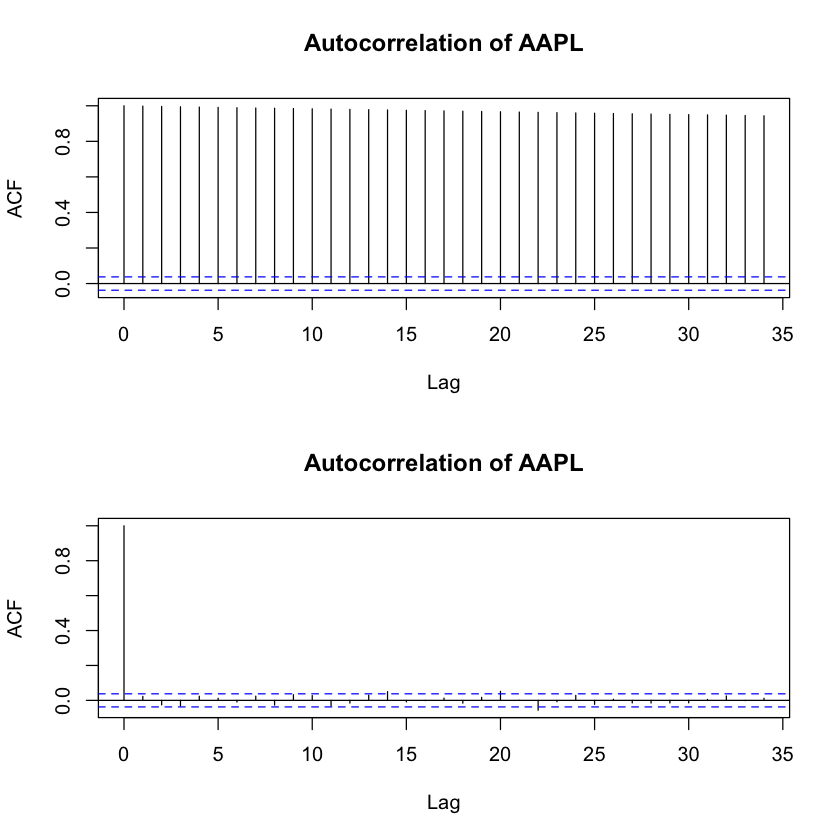Non-stationary: ACF decreases slowly (graph above) Stationary: ACF drops to zero relatively quickly (graph below)

In :
plot(diff(as.ts(AdjClosed)), main = 'AAPL Stock Prices, First Difference')In :
# APPL % Daily Returns
par(mfrow = c(3,1))
plot(APPLReturns, main = "AAPL % returns", col = "navyblue")
hist(APPLReturns , breaks = 100, col="green")
acf(APPLReturns[-1], main = "Autocorrelation plot of returns (Dependency of Returns)")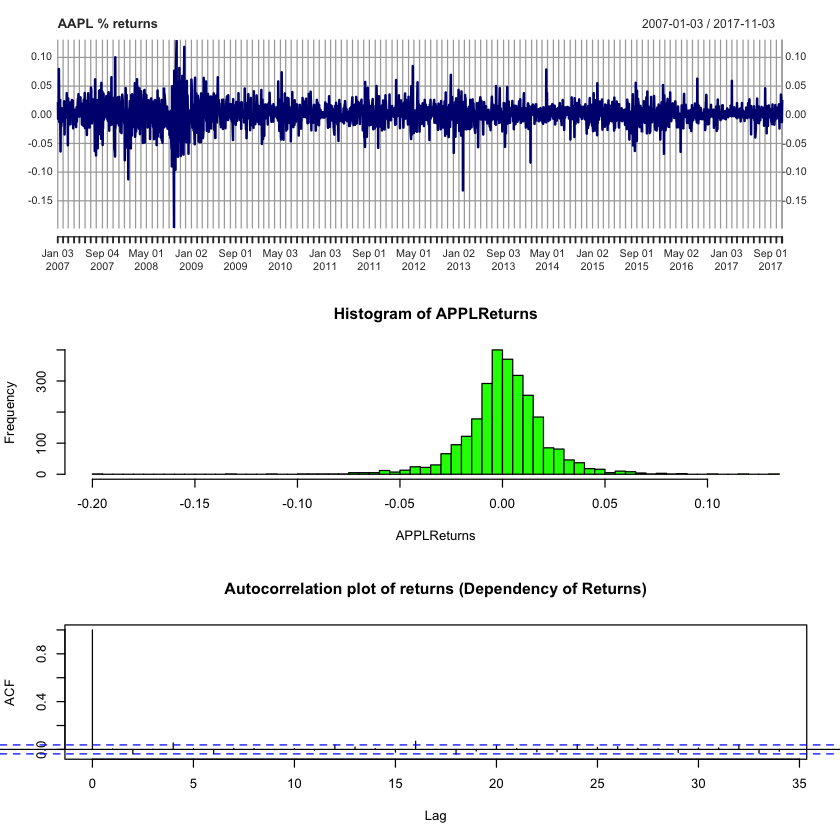AAPL % returns series is stationary as it quickly dropped to zero, and the histogram of APPL returns also show that the percentage of return is mostly concentrated around 0. The autocorrelation plot shows that today's stock price does not dependent on yesterday's stock price.

In :
# Mean Returns
meanReturns  =  mean(APPLReturns[-1])
meanReturns

0.00101597701076485
In :
# Standard Deviation of Returns
stddR =  sd(APPLReturns[-1])
stddR

0.0202007079360825
In :
# Distribution of Daily Returns
probs = c(.005, .025, .25, .5, .75, .975, .995)
distMeanReturns = quantile(APPLReturns, probs= probs, na.rm = TRUE)
distMeanReturns

0.5%
-0.0685383264032099
2.5%
-0.0418313750388579
25%
-0.00790655565717668
50%
0.00100770506469106
75%
0.0113419805352502
97.5%
0.0403593252691712
99.5%
0.0649046868398268

The median daily log return 95% confidence interval is between -0.042 and 0.040.

In :
# The Actual % Return of AAPL
(exp(meanReturns)-1) * 100

0.10164932902359

Thus, the mean daily return is 0.1016% more than the previous daily price. Although this number is small, it compounds daily at an expoential rate.

The NYSE nad NASDAQ have 252 trading days on average per year. Let's suppose we want to simulate AAPL's stock price in the next 1000 days.

In :
# Random Walk Simulation for Stock Prices
par(mfrow = c(1,1))
N = 1000 # 1000 trading days
mu = meanReturns
sigma = stddR
day =  1:N
priceInit = AAPL$AAPL.Adjusted[[length(AAPL$AAPL.Adjusted)]] # the latest daily price
# Simulate prices
set.seed(386)
price  =  c(priceInit, rep(NA, N-1))
for(i in 2:N) {
price[i] =  price[i-1] * exp(rnorm(1, mu, sigma)) # new price
}
priceSim =  data.frame(day, price)
# Visualize price simulation using ggplot
library(ggplot2)
ggplot(priceSim, aes(x=day, y=price)) +
geom_line() +
labs(y="Price",
x="Day",
title="AAPL: Simulated Prices for 1,000 Trading Days")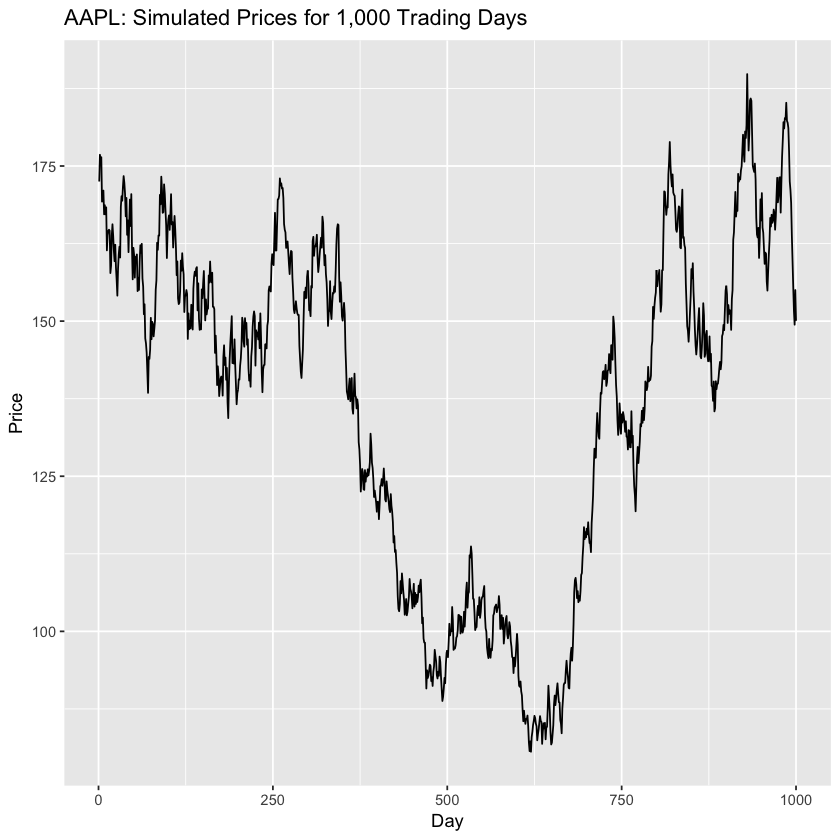This single random walk tells us that investing money in AAPL is not a good idea as there are many dips going on in this simulation over the 1000 trading days.

Read more on this random walk model : http://www.math.ucla.edu/~caflisch/181.1.03f/Lect4-5.pdf

However, the above is just a single random walk simulation -- one of many probabilistic outcomes. We can use Monte Carlo simulation to perform the above random walk process hundres or even thousands of times.

In :
# Monte Carlo Simulation
# Parameters
N = 252 # Number of Stock Price Simulations
M = 500 # Number of Monte Carlo Simulations
mu = meanReturns
sigma = stddR
day =  1:N
priceInit = AAPL$AAPL.Adjusted[[length(AAPL$AAPL.Adjusted)]]
# Simulate prices
set.seed(123)
monteCarlo =  matrix(nrow = N, ncol = M)
for (j in 1:M) {
monteCarlo[[1, j]] = priceInit
for(i in 2:N) {
monte_carlo_mat[[i - 1, j]] * exp(rnorm(1, mu, sigma))
}
}

In :
# Visualize the simulation
library(reshape2)
library(ggplot2)
df = data.frame(day, monteCarlo)
long = melt(df, id="day")  # convert to long format
ggplot(long, aes(x=day, y=value)) +
geom_line(colour="green", size=1, alpha = 0.3) +
labs(y="Simulated Stock Price",
x="Day",
title="AAPL: Simulated Prices for 252 Trading Days")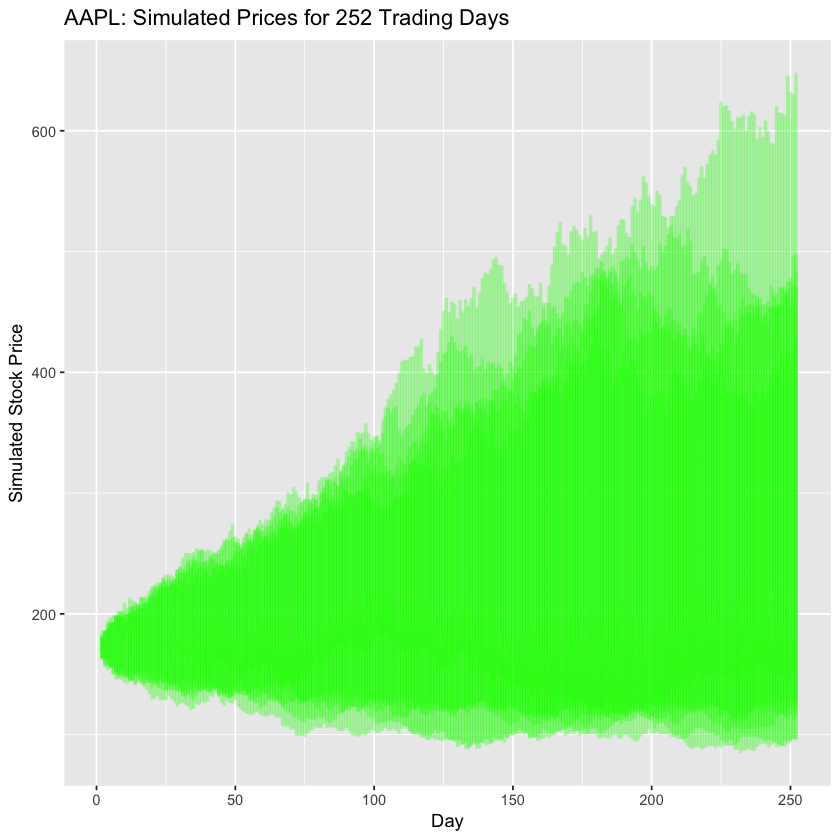In :
# Looking at the last day's stock price of each simulation
probs = c(.005, .025, .25, .5, .75, .975, .995)
dist = quantile(df$X252, probs = probs) dist  0.5% 167.25673570556 2.5% 182.340204393325 25% 204.082893481813 50% 245.210167138163 75% 354.728682086268 97.5% 438.477379848134 99.5% 446.91809809131 On the last day of the simulation, the 95% confidence interval is between \$182.34 - \$438.48, with a median estimated price of$238.73.

# Portfolio Simulation¶

Here I am going to analyze my boyfriend's portfolio. He is in the tech industy, so his investments are mostly in tech companies.

In :
 require(MASS)
require(quantmod)

# Load a few symbols into memory
getSymbols(c("AAPL", "NVDA", "BABA", "AMD", "GLW", "LMT", "RTN", "BA"))

# Plot the prices of these stocks
par(mfrow = c(4,2))
plot(AAPL[,6], main = "AAPL")
plot(NVDA[,6], main = "NVDA")
plot(BABA[,6], main = "BABA")
plot(AMD[,6], main = "AMD")
plot(GLW[,6], main = "GLW")
plot(LMT[,6], main = "LMT")
plot(RTN[,6], main = "RTN")
plot(BA[,6], main = "BA")

pausing 1 second between requests for more than 5 symbols
pausing 1 second between requests for more than 5 symbols
pausing 1 second between requests for more than 5 symbols
pausing 1 second between requests for more than 5 symbols

1. 'AAPL'
2. 'NVDA'
3. 'BABA'
4. 'AMD'
5. 'GLW'
6. 'LMT'
7. 'RTN'
8. 'BA'